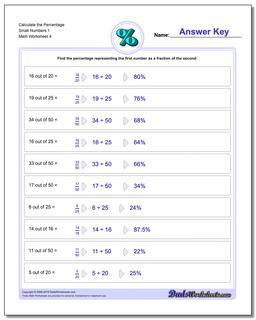PLEASE GO BACK AND USE THE BIG BLUE 'PRINT' BUTTON ON THE PAGE TO PRINT THE WORKSHEET CORRECTLY!Sorry for the trouble! The browser won't print the embedded worksheet PDF directly using the normal 'Print' command in the file menu, so you need to click the big 'Print' button to send just the worksheet and not the surrounding page to the printer.

# Math Worksheets: Percentages: Percentages: Calculate the Percentage Small Numbers 1 (Fourth Worksheet)## Calculate the Percentage Small Numbers 1 (Fourth Worksheet)

PropertyValue
DescriptionCalculate the Percentage Small Numbers 1: Percent worksheets where students are given two numbers and must determine what percentage one number is of the other number. (Fourth Worksheet)
Resource TypeWorksheet